# Construction: the Incircle of a Triangle

Compass and straight edge constructions are of interest to mathematicians, not only in the field of geometry, but also in algebra. Ancient Greek mathematicians were interested in the problem of "trisecting an angle" (splitting an arbitrary angle into three equal parts) using only a straight edge and compass. It turns out that some, but not all angles can be trisected, but no-one managed to prove that some angles could not be trisected using a straight edge and compass until the 19th Century!

However, it is possible to construct the incircle of a triangle using only a straight edge and compass.

For this construction, you will need a straight edge (ruler - but you won't be measuring anything), pair of compasses, a pencil and paper. I have drawn the pictures using the robocompass app. It's fun to play with, and you can use it to do all sorts of geometric constructions. There's a little bit of coding to learn, but a list of instructions is provided. Once you've written your little program, you can invite a few friends over, settle down in some comfy chairs, sit back, relax and enjoy the show. Be warned that, when you are using it to draw arcs, the robocompass compass point may appear to be a little away from the centre, but the drawing is actually accurate.

This construction uses the constructions for bisecting angles twice. I've gone through the steps for this construction once, but have assumed that you know what to do the second time. There's an article on bisecting an angle, if you'd like a quick refresher. You might also like to refresh your memory of drawing a perpendicular to a line from a point not on the line. There's an article you can read about that too.

## The Construction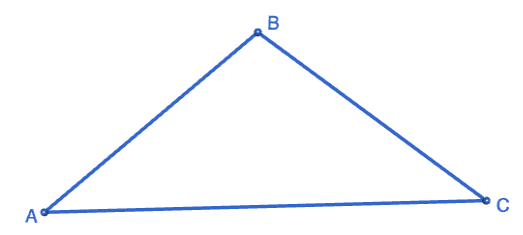Step 1: Start out by drawing the triangle $ABC$ that you want to find the incircle of. Please don't be like Sam! Use a ruler to draw the triangle.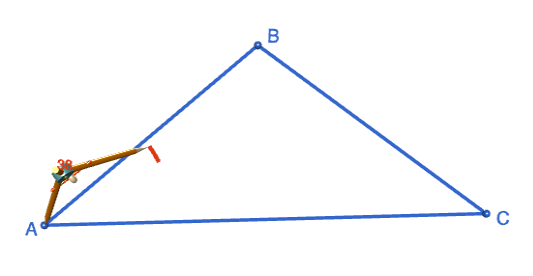Step 2: Place the tip of your pair of compasses on point $A$ (or any of the triangle's vertices), and open them out to part of the way along your line segment. You are going to draw four arcs of this radius, so make sure you keep it fixed. Draw two arcs (one on each of the adjacent sides of the triangle). Make sure your compass tip is exactly on point $A$.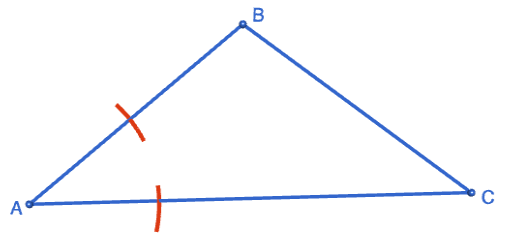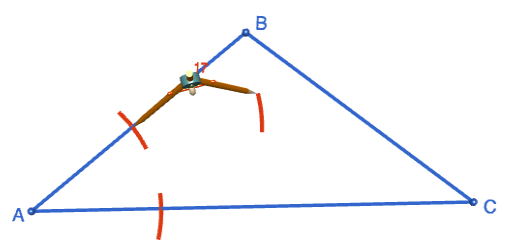Step 3: Keeping your compasses at the same radius as in the preceding step, place the tip of your pair of compasses on one of the points where the arcs from step 1 cut the sides of the triangle. Draw an arc on the inside of the triangle.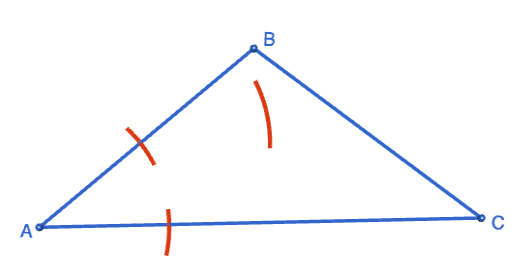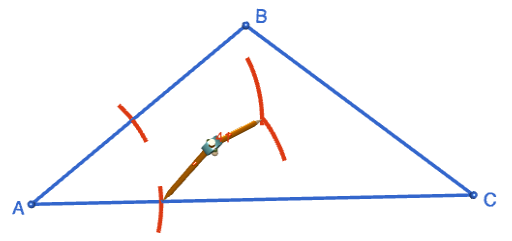Step 4: Keeping your compasses at the same radius as in the preceding step, place the tip of your pair of compasses on the other point where the arcs from step 1 cut the sides of the triangle. Draw an arc on the inside of the triangle that intersects with the arc from Step 3.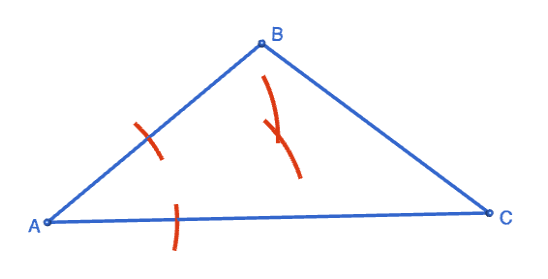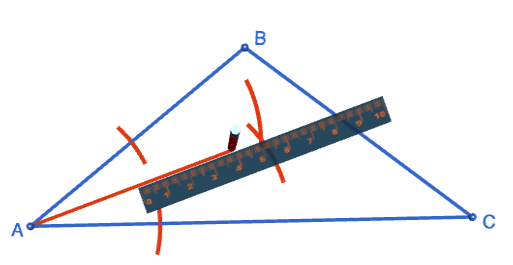Step 5: Use the straight edge (please) to draw a straight line segment from the vertex of the triangle (point A), through the point where the two arcs inside the triangle intersect. This line bisects angle $A$.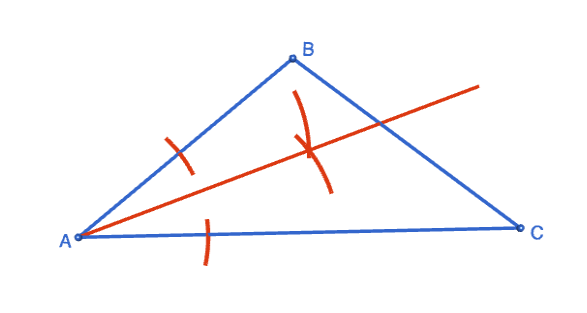Step 6: Bisect another angle of the triangle by following steps 1-5 above. The point where the two bisectors intersect will be the incentre of the triangle. Label it with an $O$. Tidy up your diagram by erasing all the arcs. If you don't, you'll get lost!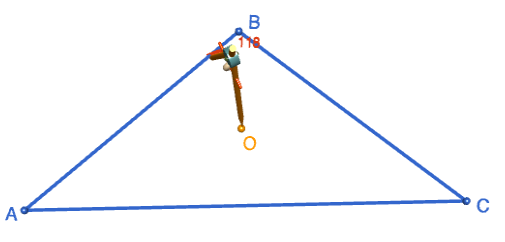Step 7: Now we need to draw a perpendicular from the incentre $O$ to one of the sides of the triangle. I've chosen side $AB$. To read about the steps I've followed, check out the article on drawing a perpendicular from a point not on a line.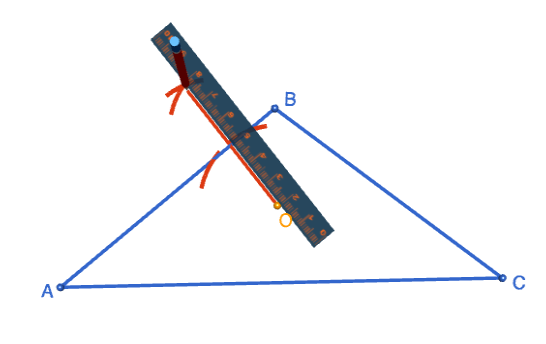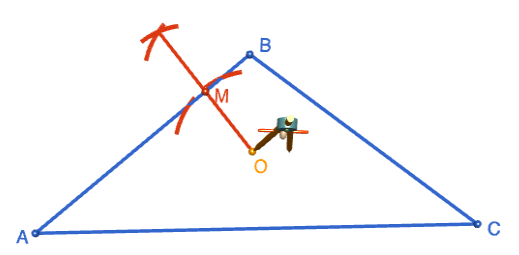Step 8: Label the point where the perpendicular cuts side $AB$ by $M$. Open out the compasses to a radius equal to the distance from $O$ to $M$, stick the tip of the compasses in at point $O$ and draw a circle. This is the incircle of the triangle.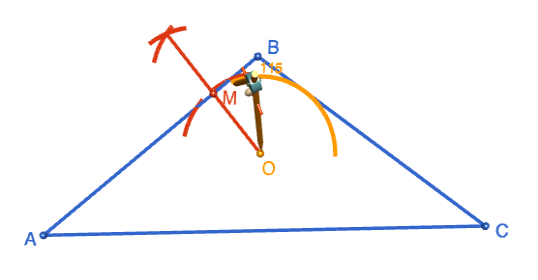That was a bit of a marathon, wasn't it? Here's the completed circle. Sit back and admire your work!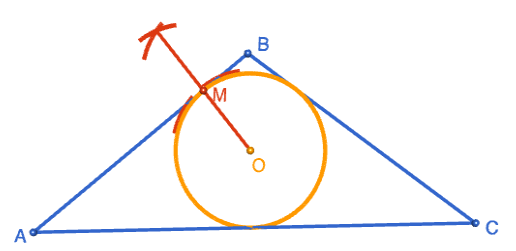### Description

This tutorial will expose you to Rulers and how to use them. We will also have a close look as set square and compass constructions. Your feedback is important to us, if you like any other topic covered under this tutorial, please do let us know.

### Audience

Year 10 or higher

### Learning Objectives

Ruler and compass constructions and more

Author: Subject Coach
You must be logged in as Student to ask a Question.